# Example Program Drafts

cancel
Showing results for
Did you mean:

## Overview

A thermistor is a type of resistor whose resistance varies significantly with temperature. Thermistors are typically desirable for applications that require a very accurate temperature measurement over a narrow temperature range. Thermistors are available for temperatures between -50°C and 100°C. Many thermistors have a sensitivity of 3-6% per °C which means that they may vary in resistance by more than ±500% of their nominal value over their full measurement range. This wide range presents a measurement challenge that can be addressed by making a half bridge measurement with a correctly chosen reference resistor.

### 1. The Measurement Circuit (Half-Bridge)

The half bridge configuration are taken from page 7 of the NI 9219 Datasheet or page 22 of the NI 9219 Getting Started Guide.  With slight modifications to this image, the figure below can be created to measure thermistors.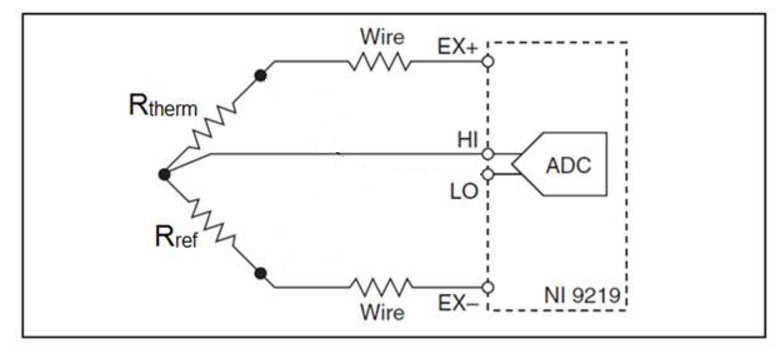Figure 1:  The thermistor, Rtherm, reference resistor, Rref, the two wires connecting to EX+ and EX-, and the wire connected to HI are external to the NI 9219. LO is internally connected to EX-.

### 2. Choosing a Reference Resistor

In order to maximize the accuracy of the measurement, the reference resistor should be chosen such that the minimum and maximum resistances are centered around the NI 9219’s input range.  That is, when the thermistor and reference resistor are placed in a voltage divider, the reference resistor should be chosen such that the minimum and maximum thermistor values will create equal, but opposite imbalances from a nominally balanced voltage divider.  This will keep the measurement error to a minimum. The temperature measurement error becomes larger (will be discussed and displayed later) as Vout approaches ground or Vex i.e. Rtherm approaches 0Ω or 1MΩ, full scale magnitude resistance.  Here’s an image to make things more clear:Figure 2:  Circuit diagram used for the derivation of the optimal reference resistor value.

The PR103J2 thermistor from US Sensor is chosen, and the range of interests is from -8°C to 65°C.  Using this sensors data sheet, we can see that -8°C corresponds to a thermistor resistance of ~50kΩ and 65°C corresponds to thermistor resistance of ~2kΩ.  Rref should be optimized to allow Rtherm to vary between 2kΩ and 50kΩ, stay inside the NI 9219's measurement range, and yield the best measurement accuracy.  In order to do this, a system of voltage divider equations is solved to find Rref.  An assumption is made that the minimum and maximum Rtherm value will provide equal but opposite imbalances from a balanced bridge in the voltage divider. A balanced bridge condition is equivalent to Vout = ½ Vex which is equivalent to Rtherm = Rref in our voltage divider.  The below equations and steps solve for Rref.

Eq. 1:Eq. 2:Eq. 1 is set equal to (-1) * Eq. 2 to solve the system of equations for Rref.

Eq. 3:The numerator on left side is multiplied with the denominator on the right side and the numerator on the right side is multiplied with the denominator of the left side to the get the result below.Rref is moved to the left side of the equation and all other components are moved to the right side and simplified to the following.Each side of the equation is divided by -2 and then the square root is taken.Plugging in Rtherm@lowerlimit =2kΩ and Rtherm@upperlimit=50kΩ the resulting Rref is 10kΩ.

Given the above thermistor resistance measurement range and target reference resistor value, the next step is to choose a reference resistor.  In order to maintain excellent accuracy, a high accuracy resistor with a very low temperature coefficient of drift should be used.  One example of such a resistor is the Vishay, Z201T 10K000 B, A, or Q for 0.1%/0.05%/0.02% resistor with 0.6 ppm/C drift.

### 3. Scaling the Data to Resistance and Temperature

The NI 9219 half bridge mode can only return data in units of V/V. Therefore, this V/V data must be scaled to resistance (Ω) and then to temperature (°C).  The scaling from V/V to Ω can be derived from the voltage divider equation.  The derivation must incorporate the knowledge that the reading returned by the NI 9219 in half bridge mode when wired in Figure 2 is equal to Vout/Vex – 0.5 V/V.

Eq. 4:Solving Eq. 4 for Rtherm yields a scaling equation for Rtherm in terms of the NI9219 Reading and Rref.

Eq. 5:

Eq. 5 can be used to scale the NI 9219's readings from V/V to Ω. Attached is a VI that allows a conversion from V/V to Ω, ratiometrictoresistance.vi. With the value of the resistance in ohms the scaling equation or table provided by the thermistor's vendor can be used to scale the data from ohms to temperature. Attached is a VI that allows a conversion from resistance to temperature (resistancetotemperture.vi) using the scaling equation provided by US Sensor.

### 4. Temperature Measurement Accuracy Calculation

The measurement accuracy of this measurement method needs to be evaluated in order to provide confidence that this is a suitable method for measuring thermistors. o First the measurement range from the thermistor and reference resistor bridge is calculated in the units that NI 9219 Half Bridge mode returns, V/V. This is done by using the voltage divider equation where Rref =10kΩ and Rtherm =1kΩ to 1MΩ to solve for Vout/Vex

For the voltage divider equation with Rref = 10kΩ, and Rtherm=10kΩ (lower limit) to 1MΩ (upper limit):

Eq. 6: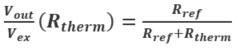The measurement range can be used to encapsulate the error of the Rref the reference resistor. For a 1% error resistor (Rreflo = 9.9kΩ and Rrefhi = 10.1kΩ) then the range can be calculated by using equation 10.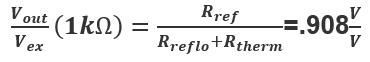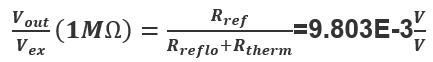The minimum and maximum values of 9.803E-3 V/V to 0.91 V/V are taken and will be used in subsequent calculations. This range is then used to calculate the measurement accuracy based on the gain, offset, and noise specification in the NI 9219’s datasheet. Equation 6 is the output of the physical bridge to which the measurement accuracy should be applied. Equation 1 is the NI 9219 Reading from software. A software subtraction is performed by 0.5 V/V in order to complete the remaining leg of the half bridge.

Eq. 7:With Eq. 7, the maximum error at the full input range (9.803E-3 V/V to 0.91 V/V error ) is calculated. The graph below shows the V/V error as a function of the measured V/V.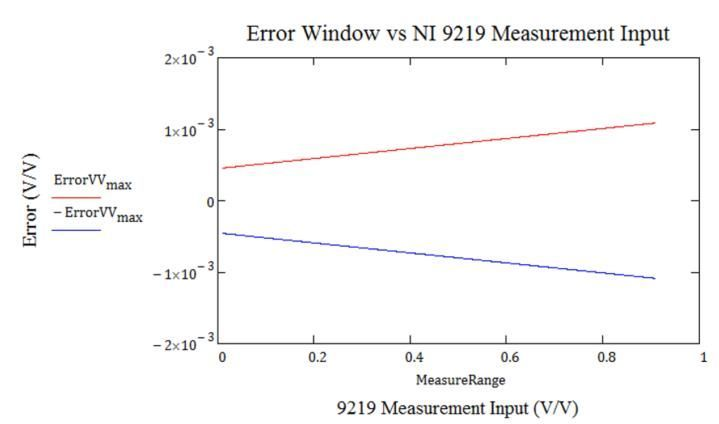Figure 3:  NI 9219 Errors in V/V.

Figure 3 shows that as the measurement range increases the error increases. Since the V/V measurement error as well as the reference resistor is known, the error in terms of resistance can be calculated with the following steps.

1. An array of the measurement range in V/V from 9.803E-3 V/V/ to 0.91V/V is created.
2. Two arrays of measurement ranges are created to account for the measurement error, measurement range ± NI9219Error. Vout/Vexhigh = measurement range + NI9219Error and Vout/Vexlow = measurement range - NI9219Error.
3. The resistance of the thermistor is calculated by solving equation 6 in terms of Rtherm.
4. Eq. 8:5. Equation 8 will yield two output arrays of Rtherm, an array of outputs for Vout/Vexhigh and Vout/Vexlow
6. From the high and low V/V measurements, due to the V/V error, the error in terms of resistance is calculated.
7. Eq. 9:The following figure is a graphical representation of equation 13, the error in terms of resistance plotted against the resistance of the thermistor.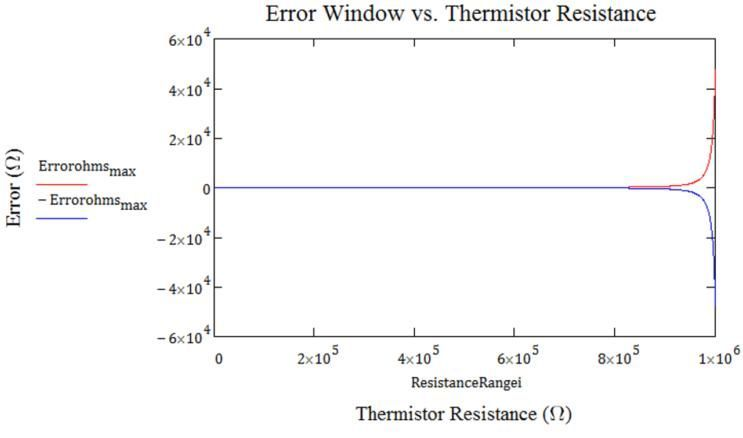Figure 4: NI 9219 Errors in Ω

Figure 4 illustrates that as the thermistor resistance increases the error increases. Ratiometrictoresistance.vi is a provided program for converting the NI 9219's readings from V/V to Ω.

From the resistance error, the temperature error can be calculated in terms of °C. Similar steps as the conversion from V/V to Ω are applied in this conversion.

1. An array of the measurement range in terms of ohms is created. The range is the same as shown in the section 4 where Rtherm =1kΩ (lower limit) to 1MΩ (upper limit).
2. Two arrays of measurement ranges in terms of resistance are created due to Resistancerangehigh=measurement range + Errorohmsmax and Resistancerangelow= measurement range-Errorohmsmax. This will result in two arrays of input resistances.
3. The temperature of the thermistor is calculated by using the Steinhart-Hart equation that models the relationship between resistance and temperature. The Steinhart-Hart coefficients are taken from the vendor's data sheet of the sensor or by contacting the vendor.
4. Eq. 10: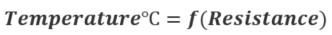5. Since there are two sets of inputs into Equation 10, Resistancerangehigh and Resistancerangelow, this will result in two sets of temperature outputs.
6. The error in terms of degrees Celsius is determined from the high and low resistance measurements (due to the resistance errors).
7. Eq. 11: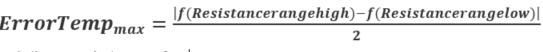From equation 11 the error in terms of degrees Celsius is graphed against the measured temperature.Figure 5: NI 9219 Errors in °C

As hinted in section 2, in choosing an appropriate reference resistor to reduce the errors for a narrow temperature range, Figure 5 displays the temperature errors and shows the increase in error at the maximum and minimum thermistor temperatures. These temperature endpoints correspond to the minimum and maximum thermistor resistances 1kΩ and 1MΩ. The maximum error of ±0.704℃ exists at the lower spectrum of the thermistor range. Within a constrained measurement window of -10℃ to 60℃, this temperature range was used to pick the reference resistor Rref, the maximum error is ~ ±0.20℃. Two VI's are attached for converting the NI 9219’s readings from V/V  to Ω (ratiometrictoresistance.vi) and then from Ω to  ℃ (resistancetotemperature.vi) for the PR103J2 thermistor. The resistancetotemperature.vi uses Steinhart-hart coefficients specific to the PR103J2 thermistor, but these coefficients can be quickly changed for other thermistors.

### 5. Measurement Errors due to thermistor Self Heating

Due to the magnitude of the excitation voltage or current used thermistors experience a self-heating effect which may lead to further inaccuracies in the measurement.  At certain temperature measurements, excitation levels, etc this will lead to additional errors and in the worst case scenario damage of the sensing element. The Dissipation Constant, provided on the sensors data sheet, can be used to calculate the temperature impact from the excitation current.

The power induced on the thermistor due to the excitation is calculated.

Eq. 12: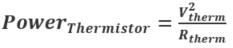Vtherm is taken from the voltage divider equation (equation 6 where Vex is on the right side of the equation). Rtherm is the resistance range of the thermistor 1kΩ to 1MΩ.Rtherm is the resistance range of the thermistor 1kΩ to 1MΩ, Rref is 10kΩ, and Vex is the excitation voltage of the NI 9219 2.5V. An expanded version of equation 12:Figure 6: NI 9219 Self Heating Errors

From the graph, the maximum self-heating error is approximately 0.156℃.  Figure 5 includes the self-heating errors.

### 6. Measurement Errors due to Lead Wire Resistance

In Figure 1 the resistance on the wires (Wire) connecting the NI 9219 to the bridge will affect the level of excitation delivered to the bridge. Limiting the amount of lead wire resistance would reduce the error but if this is not possible an understanding of how much error is created based upon the lead wire is needed. A simple approach to determine the amount of error induced by the lead wires is taken, and an assumption is made that the resistances on both legs are equal. Equation 6 is reused to determine the nominal bridge output and the bridge output due to various levels of lead wire resistance. Lead Wire resistance is denoted as Rl.  The typical output measurement from the bridge is when Rl  is equal to 0.

Eq. 13:To determine the error due to Rl we subtracted the V/V measurement due to Rl from the typical measurement.

Eq. 14: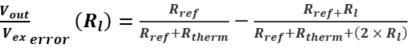The graph below plots the error for differing values of Rl and the previous V/V error window calculated in section 4 (Figure 3) against the thermistor resistance.Figure 7:  NI 9219 Measurement Errors due to the Lead Wire Resistance in V/V

An increase in the lead wire resistance directly increases the error in the measurement and dominates the measurement accuracy at higher thermistor resistances (negative temperatures). A 2.5Ω increase in the lead wire resistance from 7.5Ω to 10Ω causes the theoretical measurement error to fall outside the error window previously derived, Figure 3.   Figure 3, Figure 4, and Figure 5 error graphs do not account for any lead wire resistance errors.

### 7. Conclusion

The guidelines and derivations above provide a method of making thermistor measurements and shows the accuracy of such a measurement. The NI 9219 half-bridge mode provides a suitable method of measuring the PR103J2 thermistor with an error of <1℃.